Disclosure: This post may contain affiliate links, meaning when you click the links and make a purchase, we receive a commission.

# INDIRECT Function Excel: Get values from different sheet

While working in Excel, there are many cases where you want to refer your worksheet to a different worksheet. The topic that we will be discussing in this article is how to use Excel INDIRECT function on a different sheet where we will see the process on how the Indirect function can be used to refer a worksheet in another sheet.

## Introduction to INDIRECT function

The indirect function returns a reference from an assigned cell which contains a text string. In Excel, it is used as a reference function.

### Syntax

=INDIRECT (text_reference, [Cell_value]) which returns a genuine worksheet reference.

### Arguments

text_reference– Assigned text reference.

cell_value– It is used as an optional argument. You can specify it directly like, B1, C1 etc. It can also be defined in the R1C1 form. It is a Boolean input in Excel by default where cell value is carried as True form.

### Important Notes

• For referring another sheet, the worksheet needs to be opened.
• The result of the INDIRECT function is evaluated as real time.
• The cell_value is an optional argument and taken as Boolean input. If it is a TRUE value then the reference style will look like “=A1” this style.
• If the cell_value is a FALSE result then it will look like R1C1 style.

### Some examples of INDIRECT Function (Get a clear idea)

Look into the below picture to have some idea about the INDIRECT function.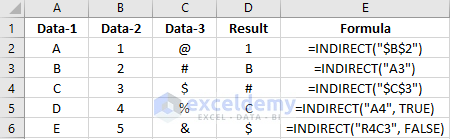## Using INDIRECT Function to refer a Worksheet

Here we are going to see how to refer another worksheet in Excel using the INDIRECT function. Create 3 worksheets titled as S1, S2, and S3 under the workbook name B1. In each S1 and S3 worksheets take some random numbers in the range of A1: C10.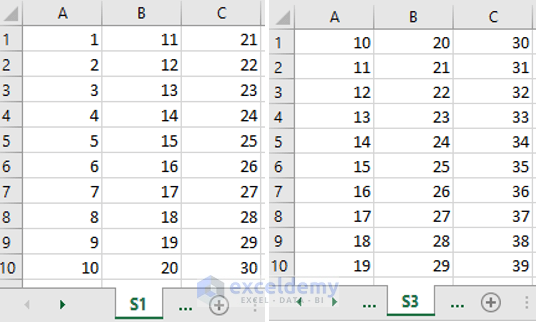We will refer to the worksheets S1 and S3 in worksheet S2. Initially, nothing is placed in worksheet S2. After inserting the data in S1 and S3 do the following things in S2.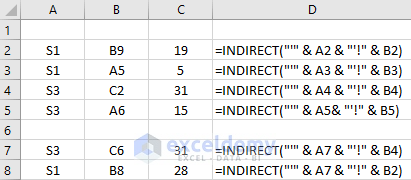In column A of Sheet S2, we will write different Sheet names. Column B will have the cell names and in different cells of column C, we will get the results based on the formula used. Let`s work on row 2 first.

In cell A2, write S1. Put a cell name say B9 in cell B2. Now in cell C2 write down the formula, `=INDIRECT("'" & A2 & "'!" & B2) `and press enter. You will get to see the result 19 in cell C2. For having a better instinct the formula is shown in cell D2.

The INDIRECT function takes the reference from cell A2 where S1 is written. A set of double quotes is used before A2 which indicates the text string. For combining the arguments, “&” is used. For separating a worksheet from a cell “!” sign is used. Here using “!” with “” we are referring sheet S1. For preventing errors, a single quote is placed inside the double-quotes. In the end, B2 is written which specify the location of the cell. After the formula is placed in cell C2 you will get the result 19 as the cell value of B9 in Sheet S1 is 19.

Insert the formula for different sheets and for different scenarios, you will get different results that you want.

In cell C7 and C9, the INDIRECT formula refers to the sheet S3 and S1 respectively. But in cell D7 the location of the cell is given as B4. In B4 of Sheet S2, C2 is written. The cell value of cell C2 in Sheet S3 is 31. So, in cell C7 we will get the result 31.

## Using INDIRECT Function to refer a Workbook

For referring a workbook in another workbook, first, make a new workbook titled as B2. Now in cell A2, A3 and A4 of workbook B2.xlsx write B1.xlsx, S5, and B4 respectively.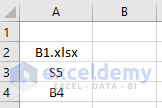Now, let`s say you want to find out the total sales of sheet S5 of workbook B1.xlsx. Write Totals Sales in cell A6 of workbook B2.xlsx. Write down the formula `=INDIRECT("'[" & A2 & "]" & A3 & "'!" & A4) `in cell B6 and press Enter. Now, you will see the result of cell B4 of worksheet S5 which is under the Workbook B1.xlsx in the worksheet Sheet1 of workbook B2.xlsx.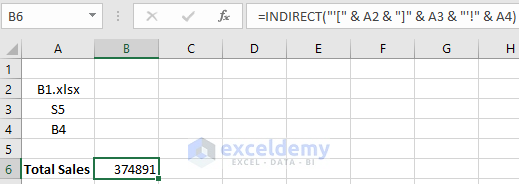## Numeric Calculation after referring a worksheet using the INDIRECT Function

Let`s say you want to calculate the SUM result of some values of a worksheet by referring it to another worksheet. To do this, make a new worksheet named S4. In cell A2, write down S1. Type the formula, `=SUM(INDIRECT("'"&A2&"'!"&"A1:A4"))` in cell B2 and you will get the result 10 in cell B2. Here the formula indicates the sheet S1 as S1 is written in cell A2. The defined range here is A1: A4. So, the formula will select the range A1: A4 and will calculate the SUM result of the values placed in those cells.

Again, the formula,`=SUM(INDIRECT("'"&A3&"'!"&"C1:C4"))` will return the value 126 in cell B3.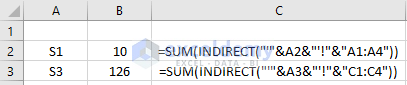## Using Excel INDIRECT Function with Data Validation

Let`s work on an example where total and average sales of years 2016, 2017, and 2018 are given in three different sheets. The sheets’ name is defined by its years. We want to find the total sales amount and average sales amount for each year on a different sheet.

To perform this, make a new worksheet named S5. Now, after selecting cell B2 click on to the Data Validation option under the Data tab. In the Data Validation dialogue box do the following and press OK.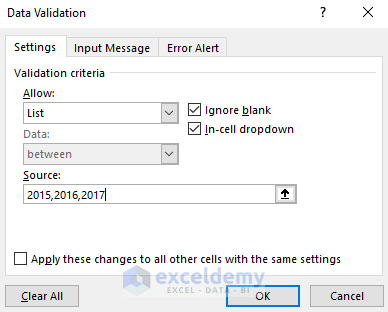Write Total sales and Average Sales in cell A4 and cell A5. Now in cell B4 write the formula,`=SUM(INDIRECT("'"&B2&"'!"&"B2:B13"))` It will calculate the Total sales of each year. In cell B5, write down the formula, `=AVERAGE(INDIRECT("'"&B2&"'!"&"B2:B13"))` for calculating the average cells. The formulas are shown in cell C4 and C5 for clearing confusions.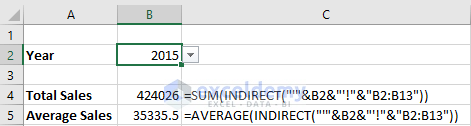Now by changing the year in cell B2, you will see the values change every time. Here we defined sheet names with data validation in cell B2. The two formulas take the reference from cell B2. So, by changing the year in cell B2 every time, the values of cell B4 and B5 changes.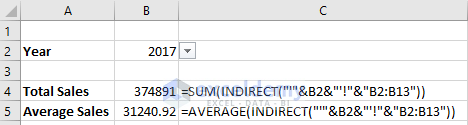## Conclusion

As you can see it is quite easy to refer a worksheet in another sheet by using the INDIRECT function. We can even perform many numeric calculations. Hope this article will find your interest. Don`t hesitate to ask any question.

## Related Articles

Hello!

Welcome to my Profile. Here I will be posting articles related to Microsoft Excel. I have completed my BSc in Electrical and Electronic Engineering from American International University-Bangladesh. I am a diligent, goal-oriented engineer with an immense thirst for knowledge and attitude to grow continuously. Continuous improvement and life-long learning is my motto.

1 Comment
1. ReplyAbdelrahman Aug 20, 2018 at 4:36 AM

Thank you for sharing.
Why it cannot work between different workbooks if is closed?
Is there any work around to get a data from a workbook even if it is closed??
Thank you again..